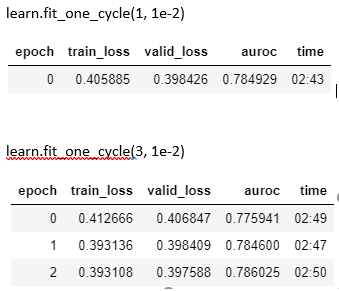# Why the first epochs are worse?

Hi, maybe this is a dumb question but: why when you increase the number of epochs the first epochs are worse? E.g:Both were ran using the same seeds, determinisc option was active, and all that stuff. Why the first epoch is much worse when you fit for more epochs?

Thanks!

So I think this is explained better than I can in the course (I think in lesson 3).

Essentially the fit_one_cycle method starts with a really high learning and then reduces it. This allows the algorithm to explore more of the parameter space (try a greater range of weights out) before zoning in on a better solution where we then decrease the learning rate. If you plot the learning rate using the learning rate recorder for both runs it will be easier to understand what I mean.

1 Like

Thanks @cbparikh, I haven’t took lesson 3 yet, maybe I will come back with more questions then!

1 Like